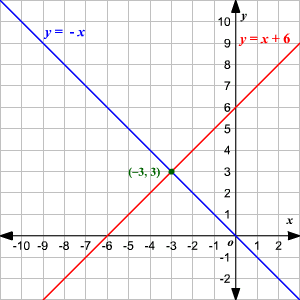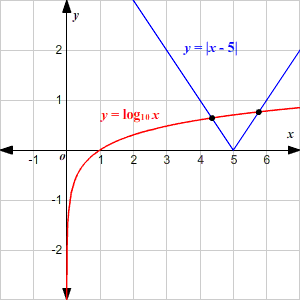# Functions: Graphs and Intersections

Suppose $f\left(x\right)$ and $g\left(x\right)$ are two functions that take a real number input, and output a real number.

Then the intersection points of $f\left(x\right)$ and $g\left(x\right)$ are those numbers $x$ for which $f\left(x\right)=g\left(x\right)$ .

Sometimes the exact values can be easily found by solving the equation $f\left(x\right)=g\left(x\right)$ algebraically.

Example 1:

What are the points of intersection of the functions $f\left(x\right)$ and $g\left(x\right)$ if $f\left(x\right)=x+6$ and $g\left(x\right)=-x$ ?

The intersection points of $f\left(x\right)$ and $g\left(x\right)$ are those numbers $x$ for which $f\left(x\right)=g\left(x\right)$ .

That is, $x+6=-x$ .

Solve for $x$ .

$\begin{array}{l}x+6=-x\\ 2x+6=0\\ 2x=-6\\ x=-3\end{array}$

Now, you can use the value of $x$ to find the corresponding $y$ -coordinate of the point of intersection.

Substitute the value of $x$ in any of the two functions.

$\begin{array}{l}g\left(-3\right)=-\left(-3\right)\\ \text{\hspace{0.17em}}\text{\hspace{0.17em}}\text{\hspace{0.17em}}\text{\hspace{0.17em}}\text{\hspace{0.17em}}\text{\hspace{0.17em}}\text{\hspace{0.17em}}\text{\hspace{0.17em}}\text{\hspace{0.17em}}\text{\hspace{0.17em}}\text{\hspace{0.17em}}\text{\hspace{0.17em}}\text{\hspace{0.17em}}\text{\hspace{0.17em}}=3\end{array}$

The equations can also be solved graphically by plotting the two functions on a coordinate plane and identifying the point of intersection of the two.In other cases, the exact values can be hard to find. You may need to use technology to estimate them.

Example 2:

Find the point(s) of intersection of the two functions.

$\begin{array}{l}f\left(x\right)=|\text{\hspace{0.17em}}x-5\text{\hspace{0.17em}}|\\ g\left(x\right)=\mathrm{log}x\end{array}$

Here, solving algebraically is not so easy .The solutions to the equation $|x-5|=\mathrm{log}x$ are not nice-looking rational numbers.

Graph the functions on a coordinate plane .You can use a graphing utility to find that the coordinates of the intersection points are approximately $\left(4.36,0.64\right)$ and $\left(5.76,0.76\right)$ .